# Measurement and Financial Mathematics | The Ultimate Guide to NESA’s Maths Reference Sheet

Make the most of NESA Maths Reference Sheet and test your maths skills with these sample questions and explanations for measurement and financial maths!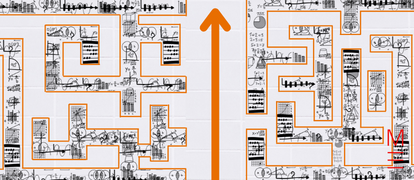The NESA Maths Reference Sheet is a great resource… if you know how to use it! Navigate measurement and financial mathematics with our Ultimate NESA Maths Reference Sheet Guide.

While memorisation has its place in learning, Matrix recommends that students learn to derive their responses and learn how to apply these formulae correctly. As a bonus, we’ve included a nifty HSC Maths Cheatsheet for you to download and print out!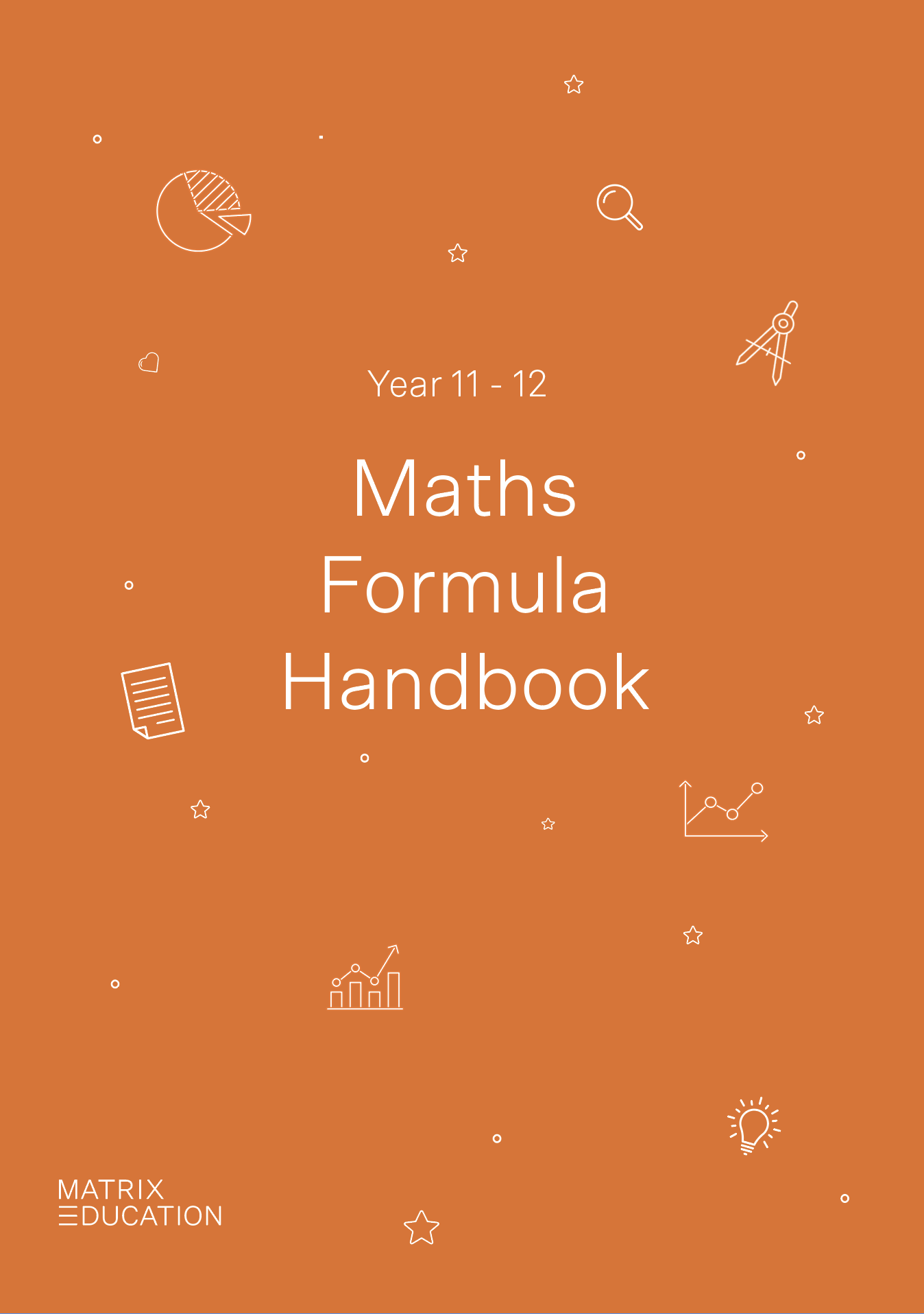A free pocket-sized Maths handbook, so you're prepared.

All the key Maths formulas you need to revise, in one foldable cheatsheet.

Click on the following formulas to see what they mean and apply them to a practice question!

## Measurement

### Length

 Use Formula Variables Arc length of a sector (using θ in degrees) $$l=\frac{\theta}{360}\times2\pi r$$ \begin{align*} l&= \text{arc length}\\ \theta &= \text{angle of sector in degrees}\\ r &= \text{radius}\\ \end{align*}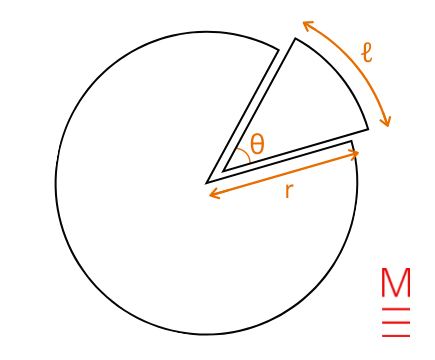Example 1:

Find the length of the minor arc AB, such that the length of radius OA is 30 cm. Leave your answer as an exact value.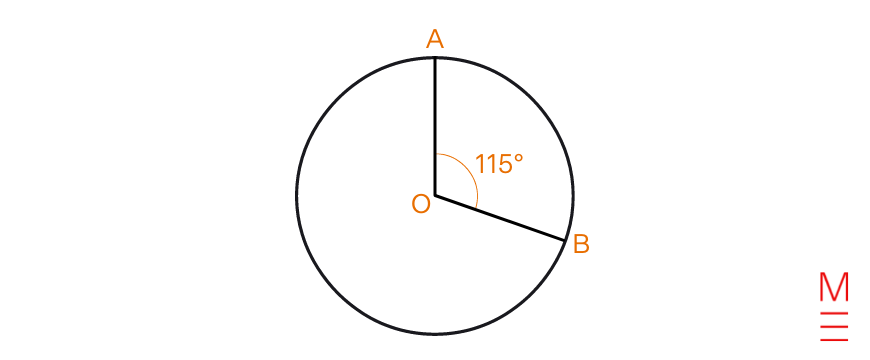Solution 1:

 Substitute $$\theta = \text{115}$$ and $$r = \text{30}$$ into $$l=\frac{\theta}{360}\times2\pi r$$: \begin{align*} l &=\frac{115}{360}\times2\pi \times 30\\ &= \frac{115}{6} \pi \\ ∴ \text{Length of the minor arc AB} &= \frac{115}{6} \pi \text{ cm}\\ \end{align*}

### Area

 Use Formula Variables Area of a sector $$A= \frac{\theta}{360} \times \pi r^2$$ \begin{align*} A&= \text{area of sector}\\ \theta &= \text{angle of sector in degrees}\\ r &= \text{radius}\\ \end{align*}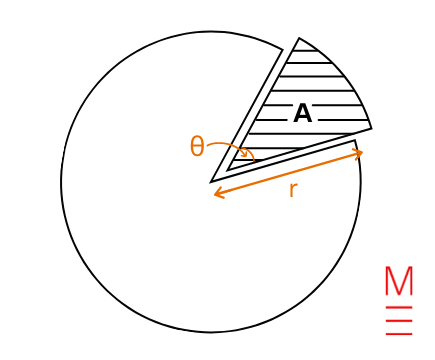Example 2:

Given that the following circle has a radius of 5 m, find the area of the major sector AOB. Leave your answer as an exact value.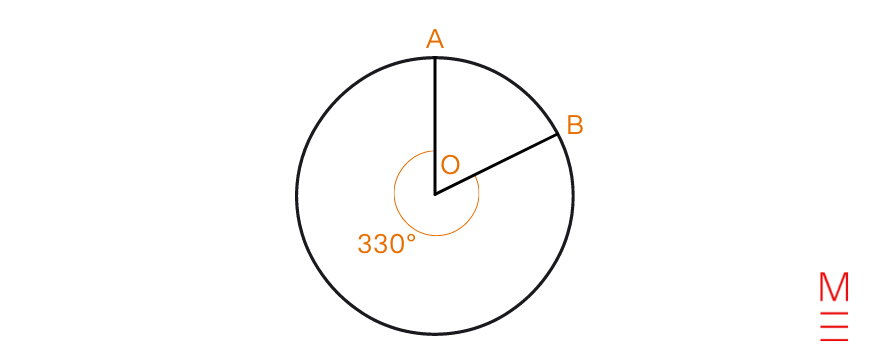Solution 2:

 Substitute $$\theta=330$$ and $$r=5$$ into $$A= \frac{\theta}{360} \times \pi r^2$$: \begin{align*} A &= \frac{330}{360} \times \pi \times {5}^2 \\ &= \frac{275}{12} \pi \\ ∴ \text{Area of the major sector AOB} &= \frac{275}{12} \pi \text{ m}^2\\ \end{align*}

 Use Formula Variables Area of a trapezium $$A=\frac{h}{2} (a+b)$$ \begin{align*} A&= \text{area of trapezium}\\ h &= \text{perpendicular height}\\ a, \ b &= \text{lengths of the parallel sides}\\ \end{align*}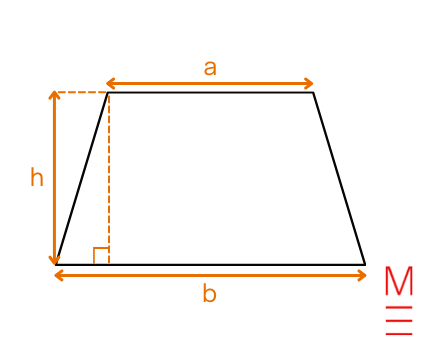Example 3:

What is the area of the shape below? Note that all the measurements were made in millimetres.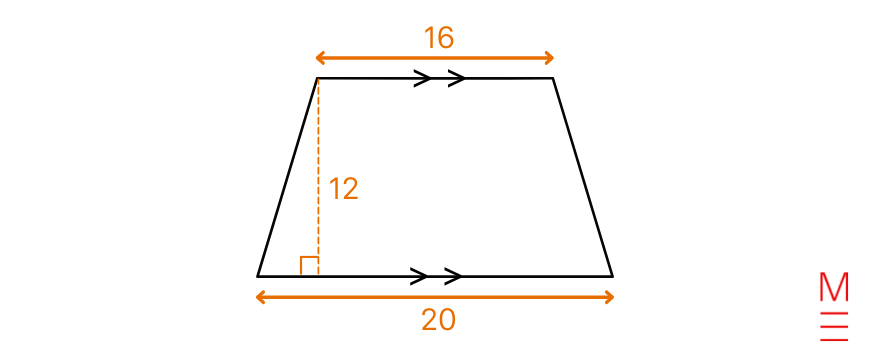Solution 3:

 Substitute $$h=12$$, $$a=16$$ and $$b=20$$ into $$A=\frac{h}{2} (a+b)$$: \begin{align*} A &= \frac{12}{2} (16+20) \\ &= 216 \\ ∴ \text{Area} &= 216 \text{ mm}^2\\ \end{align*}

### Surface area

 Use Formula Variables Surface area of a cylinder $$A=2 \pi r^2 + 2 \pi r h$$ \begin{align*} A&= \text{surface area of cylinder}\\ h &= \text{height}\\ r &= \text{radius of circular base}\\ \end{align*}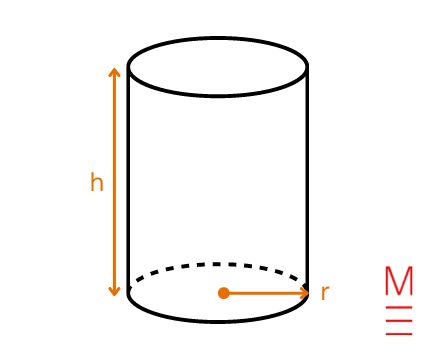Example 4:

Gemma is designing a can for her new spaghetti recipe. In order to figure out how much pink paint she needs to completely cover the can, she needs to know what the total surface area of the cylindrical can is.

Knowing that the dimensions below are given in centimetres, what is the surface area of Gemma’s can? Leave your answer as an exact value.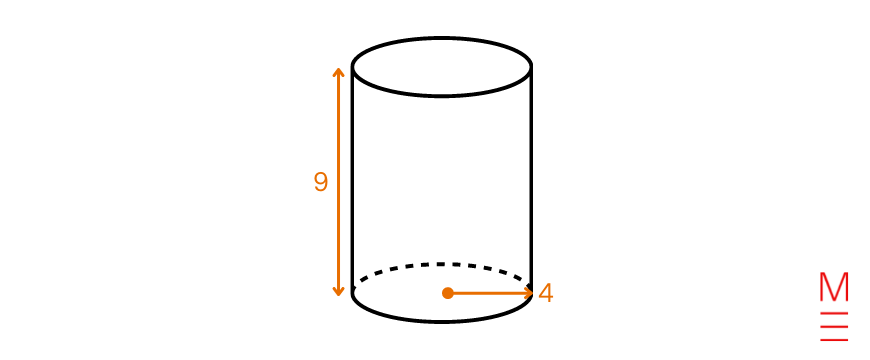Solution 4:

 Substitute $$h=9$$ and $$r=4$$ into $$A=2 \pi r^2 + 2 \pi r h$$: \begin{align*} A &=2 \pi \times 4^2 + 2 \pi \times 4 \times 9 \\ &= 32 \pi + 72 \pi \\ &= 104 \pi \\ ∴ \text{Surface area of can} &= 104 \pi \text{ cm}^2\\ \end{align*}

 Use Formula Variables Surface area of a sphere $$A = 4 \pi r^2$$ $$r = \text{radius}$$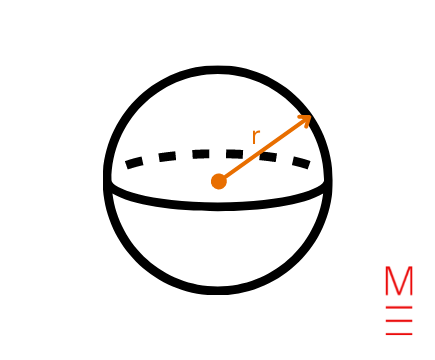Example 5:

Biological cells with larger surface area to volume ratios are able to more efficiently diffuse oxygen into the cell and waste material out.

If we approximate a cell to be the shape of a sphere, what is the surface area of a cell with a diameter of 0.07 mm? Leave your answer as an exact value.

Solution 5: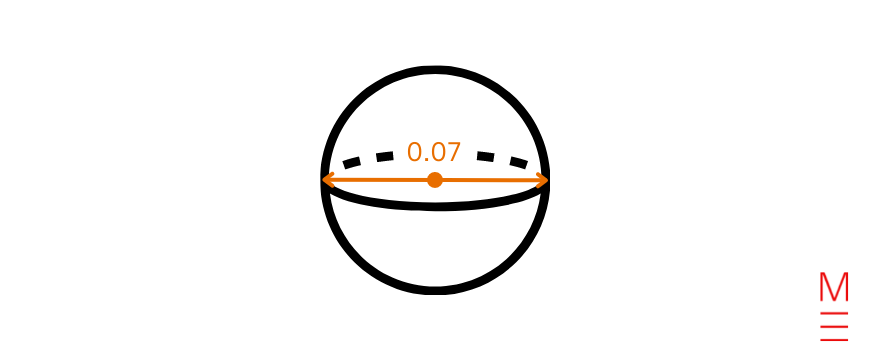Substitute $$r= \frac{0.07}{2}$$ (since the radius is half of the diameter) into $$A = 4 \pi r^2$$: \begin{align*} A &= 4 \pi \times \left( \frac{0.07}{2} \right)^2 \\ &= 4 \pi \times 0.035^2 \\ &= 0.0049 \pi \\ ∴ \text{Surface area of cell} &= 0.0049 \pi \text{ mm}^2\\ \end{align*}

### Volume

 Use Formula Variables Volume of a pyramid/cone $$V= \frac{1}{3}Ah$$ \begin{align*} V &= \text{volume of pyramid/cone}\\ A &= \text{base area}\\ h &= \text{height}\\ \end{align*}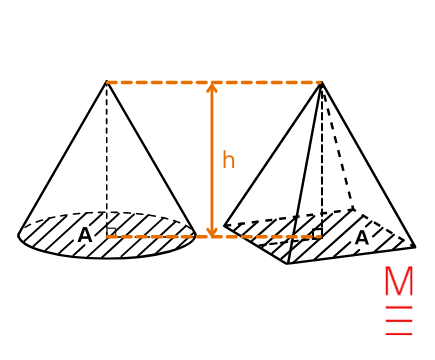Example 6:

Ricky is planning her birthday party. She wants to prank her friends by putting water balloons inside all of the party hats.

Assuming that the party hats are a perfect cone with a radius of 5 cm and a height of 12 cm, how much water does she need to fill one party hat? Leave your answer as an exact value.

Solution 6: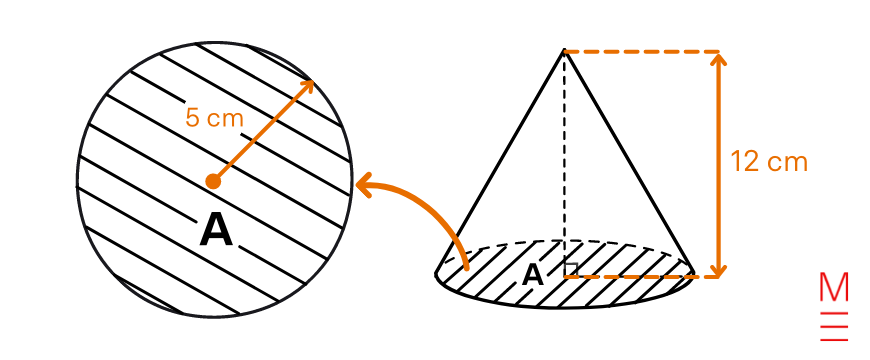To find the area of the circular base, substitute $$r=5$$ into $$A= \pi r^2$$: \begin{align*} A &= \pi \times 5^2  \\ &= 25 \pi \\ ∴ \text{Base area} &= 25 \pi \text{ cm}^2\\ \end{align*}   Substitute $$A= 25 \pi$$ and $$h=12$$ into $$V= \frac{1}{3}Ah$$: \begin{align*} V &=\frac{1}{3} \times 25 \pi \times 12 \\ &= 100 \pi \\ ∴ \text{Volume of water needed} &= 100 \pi \text{ cm}^3\\ &= 100 \pi \text{ mL}\\ \end{align*}

 Use Formula Variables Volume of a sphere $$V= \frac{4}{3} \pi r^3$$ $$r = \text{radius}$$Example 7:

Gordon is making meatballs. Assuming that 1 cm3 = 1 gram, how much mince does he need to make a perfectly spherical meatball with a diameter of 3 cm? Leave your answer as an exact value.

Solution 7:

 Substitute $$r= \frac{3}{2}$$ (since the radius is half of the diameter) into $$V= \frac{4}{3} \pi r^3$$: \begin{align*} V &= \frac{4}{3} \pi \left( \frac{3}{2} \right) ^3 \\ &= \frac{9}{2} \pi \\ ∴ \text{Amount of mince needed} &= \frac{9}{2} \pi \text{ cm}^3\\ &= \frac{9}{2} \pi \text{ g}\\ \end{align*}

## Financial Mathematics

 Use Formula Variables Compound interest $$A= P(1+r)^n$$ \begin{align*} A &= \text{value of investment at the end of the n}^{th} \text{ period}\\ P &= \text{principle (initial) amount}\\ r &= \text{compound interest rate per period — expressed as a decimal}\\ n &= \text{number of time periods}\\ \end{align*}

Example 8:

Jacky’s bank is offering her a fantastic interest rate of 3% per annum, compounded every three months. If she deposits 12 360 into her savings account today and resolves to not take away from or add to these funds, what will be her savings account balance in 12 months time? Provide your answer to the nearest two decimal places. Solution 8:  \begin{align*} A & = \text{Jacky’s savings account balance in 12 months time: this is what we are trying to find!} \\ P & = 12 \ 360 \\ \end{align*}The interest rate of 3% per year is compounded every three months. So, we need to divide the 3% interest rate by the number of three month periods in 12 months to find the compound interest rate per three month period:\begin{align*} r & = \frac{3 \text{%}}{ \frac{12}{3} } \\ & = \frac{0.03}{4} \\ ∴r & = 0.0075 \\ \end{align*}Since the compounding period is three months and Jacky plans to leave her savings account balance as it is for 12 months:\begin{align*} n & = \frac{12}{3} \\ ∴n & = 4 \\ \end{align*}Hence, substitute $$P=12 \ 360$$, $$r=0.0075$$ and $$n=4$$ into $$A= P(1+r)^n$$:\begin{align*} A &= 12 \ 360 \times (1+0.0075)^4 \\ &= 12 \ 360 \times 1.0075^4 \\ &= 12 \ 734.99 \text{ (to the nearest two decimal places)} \\ ∴ \text{Jacky’s savings account balance in 12 months time} &=12 \ 734.99\\ \end{align*}

## Sequences and series

Note: A series is the sum of the elements in a sequence.

### Arithmetic Progressions

In an arithmetic progression, each term is determined by adding a constant to the preceding term.

 Use Formula Variables Arithmetic sequence $$T_n = a+(n-1)d$$ \begin{align*} T_n &= \text{n}^{th} \text{ term of the sequence}\\ S_n &= \text{sum of the first n terms of the sequence}\\ \\ n &= \text{position of the term}\\ a &= \text{first term: }T_1\\ d &= \text{common difference between terms}\\ l &= \text{last term being added: }T_n\\ \end{align*} Arithmetic series $$S_n=\frac{n}{2} [2a+(n-1)d] = \frac{n}{2}(a+l)$$

Example 9:

Consider the following arithmetic sequence:

$$3, 15, 27, 39…$$

What is the 23rd term of this sequence?

Solution 9:

 Substitute $$n=23$$, $$a=3$$ and $$d=12$$ into $$T_n = a+(n-1)d$$ \begin{align*} T_{23} & = 3+(23-1) \times 12\\ &= 3 + 22 \times 12 \\ &= 267 \\ ∴ \text{23}^{rd} \text{ term: } & 267\\ \end{align*}

Example 10:

The sum of the first 16 terms of an arithmetic series is 632. Given the first term of the series is 2, write down the first 4 terms of the series.

Solution 10:

 Substitute $$S_n=632$$, $$n=16$$ and $$a=2$$ into $$S_n=\frac{n}{2} [2a+(n-1)d] = \frac{n}{2}(a+l)$$ \begin{align*} 632 & =\frac{16}{2} [2 \times 2 +(16-1)d]\\ 632 &= 8 \times (4+ 15d) \\ 632 &= 32+ 120d \\ 600 &= 120d \\ ∴ d &= 5\\ \end{align*} \begin{align*} T_1 & = a = 2 \text{ (given)}\\ \text{Since } T_n & = a+(n-1)d \text{:}\\ T_2 &= 2 + 5 \\ &= 7 \\ T_3 &= 2+ 2 \times 5 \\ &= 12 \\ T_4 &= 2 + 3 \times 5 \\ &= 17 \\ \text{∴ First 4 terms of the series: }& 2 + 7 + 12 +17\\ \end{align*}

### Geometric Progressions

In a geometric progression, each term is found by multiplying the previous term by a constant.

 Use Formula Variables Geometric sequence $$T_n=ar^{n-1}$$ \begin{align*} T_n &= \text{n}^{th} \text{ term of the sequence}\\ S_n &= \text{sum of the first n terms of the sequence}\\ \\ n &= \text{number of terms being added}\\ a &= \text{first term: }T_1\\ r &= \text{common ratio between terms}\\ \end{align*} Geometric series $$S_n=\frac{a(1-r^n)}{1-r}=\frac{a(r^n-1)}{r-1}, \ r≠1$$ Limiting sum of a geometric series $$S= \frac{a}{1-r}, \ |r|<1$$

Example 11:

A basketball player is bouncing a ball. On each bounce, the ball reaches a height that is 85% of its previous height. The total distance that the ball has travelled just before they make contact with the ball for the 8th time is 6 metres.

a) At what height did the basketball player begin bouncing the ball? Give your answer in metres to the nearest three decimal places.

b) Hence or otherwise, find the total distance that the ball travels if the basketball player continues bouncing the ball until it eventually does not lift off the ground. Give your answer in metres to the nearest two decimal places.

Solution 11 a):

 $$\text{Let }x = \text{initial bounce height of the ball}$$ Let’s consider what this problem would look like visually.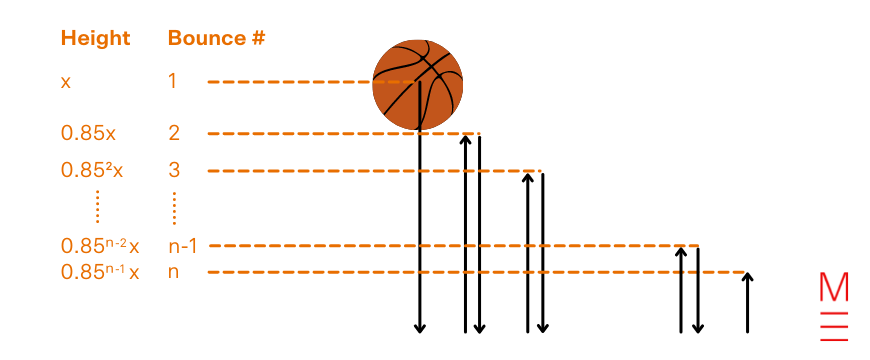The height of each bounce conveniently forms a geometric sequence where the common ratio r=0.75 and the first term a=x. Hence, $$T_n=x \times 0.75^{n-1}$$ However, the distance that the ball travels is not a simple geometric series. As you can see in the diagram above, apart from the first bounce and final n bounce (where the ball only travels downwards or upwards), the ball travels twice the distance of the bounce height as it travels up and down. Hence, we can find the total distance d travelled by the ball before the nth bounce: \begin{align*} d &=  2 \times [\text{sum of the heights of the 1st, 2nd, 3rd, …, (n-2)th, (n-1)th and nth bounce}] -[\text{height of the 1st bounce}] – [\text{height of the nth bounce}]\\ &= 2 \times (T_1+T_2+T_3+…+T_{n-2}+T_{n-1}+T_n) – T_1 – T_n\\ ∴d &= 2 \times S_n – T_1 – T_n\\ \end{align*} In this question, we are given the total distance travelled by the ball before the 8th bounce is 6 metres. So, substitute d=6 and  n=8 into $$d = 2 \times S_n – T_1 – T_n$$: \begin{align*} 6 &= 2 \times S_8 – T_1 – T_8 \text{…………. (equation 1)}\\ \end{align*} To find S8, substitute $$a=x$$, $$n=8$$ and $$r=0.75$$ into $$S_n=\frac{a(1-r^n)}{1-r}$$: \begin{align*} S_8 &=\frac{x(1-0.75^8)}{1-0.75}\\ &= 4[x(1-0.75^8)] \\ \end{align*} To find T8, substitute $$a=x$$ and $$n=8$$ into $$T_n=ar^{n-1}$$: \begin{align*} T_8 &= x \times 0.75^{8-1} \\ &= x \times 0.75^7 \\ \end{align*} Hence, we can substitute $$S_8=4[x(1-0.75^8)]$$, $$T_1= x$$ and $$T_8= x \times 0.75^7$$ into equation 1: $$6 = 2 \times S_8 – T_1 – T_8$$: \begin{align*} 6 &= 2 \times 4[x(1-0.75^8)] – x – x \times 0.75^7\\ 6 &= 8[x(1-0.75^8)] – x – x \times 0.75^7\\ 6 &= x[8(1-0.75^8) – 1 – 0.75^7] \\ x &= \frac{6}{8(1-0.75^8) – 1 – 0.75^7} \\ x &= 0.989 \text{ (rounded to 3 decimal places)} \\ ∴ \text{Height at which player began bouncing ball} &= 0.989 \text{ m}\\ \end{align*}

Solution 11 b):

 In part a), we found that the total distance d travelled by the ball is $$d = 2 \times S_n – T_1 – T_n$$. Need a refresher? Click here to scroll up to the explanation in part a). In part a), we were interested in the distance that the ball travels before the 8th bounce. So, we used $$n = 8$$. In part b) though, we are trying to find how far the ball can travel if it was allowed to bounce forever. So here, we let $$n = ∞$$. Substitute $$n = ∞$$ into $$d = 2 \times S_n – T_1 – T_n$$: \begin{align*} d &= 2 \times S_∞ – T_1 – T_∞\\ \end{align*} Since: \begin{align*} T_1 &=a \\ &=0.989 \text{ (from part a)}\\ S_∞ &= \frac{a}{1-r}, \  \text{ where a=0.989, r=0.75 and|r|<1} \\ &=  \frac{0.989}{1-0.75}\\ & = 3.956\\ T_∞ &= 0 \text{ (the ball does not lift off the ground on the ‘last’ bounce)}\\ \end{align*} \begin{align*} ⇒ d &= 2 \times S_∞ – T_1 – T_∞\\ &= 2 \times 3.956 – 0.989 – 0\\ &= 6.92 \text{ (rounded to 2 decimal places)}\\ ∴ \text{Total limiting distance} &= 6.92 \text{ m} \\ \end{align*}

Review ‘Series and Sequences’ and download your free worksheet here.